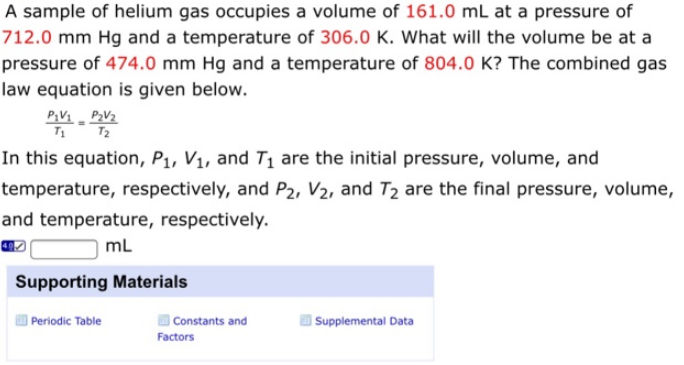# Problem: A sample of helium gas occupies a volume of 161.0 mL at a pressure of 712.0 mm Hg and a temperature of 306.0 K. What will the volume be at a pressure of 474.0 mm Hg and a temperature of 804.0 K? The combined gas law equation is given below. P1V1/T1 = P2V2/T2 In this equation, P1, V1, and T1 are the initial pressure, volume, and temperature, respectively, and P2, V2, and T2 are the final pressure, volume, and temperature, respectively.

###### FREE Expert Solution
84% (358 ratings)###### Problem Details

A sample of helium gas occupies a volume of 161.0 mL at a pressure of 712.0 mm Hg and a temperature of 306.0 K. What will the volume be at a pressure of 474.0 mm Hg and a temperature of 804.0 K? The combined gas law equation is given below.

P1V1/T1 = P2V2/T2

In this equation, P1, V1, and T1 are the initial pressure, volume, and temperature, respectively, and P2, V2, and T2 are the final pressure, volume, and temperature, respectively.